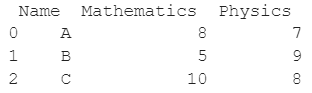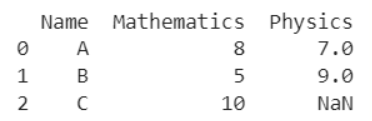# How To Check If Any Value Is NaN In A Pandas DataFrame?

A DataFrame is the primary data structure of the Pandas library and is commonly used for storing and working with tabular data. A common operation that could be performed on such data is to check if any value is NaN in the dataframe in order to deduce information from it.

To start working with Pandas, we first need to import it into Python code:

``import pandas as pd``

## Running Example

Let us understand this operation with the help of an example. Consider the following DataFrame containing 3 students with names A, B, and C and their corresponding marks (out of 10) for two subjects, Mathematics and Physics. Let this be dataframe df1Code snippet for generating the above DataFrame :

### Python 3 Code :

``````import pandas as pd
# Dictionary for our data
data1 = {'Name' : ['A', 'B', 'C'], 'Mathematics' : [8, 5, 10], 'Physics' : [7, 9, 8]}
# DataFrame for the dictionary
df1 = pd.DataFrame(data1)
# Printing the DataFrame
print(df1)``````

Here, data1 is a dictionary we created to initialize the DataFrame. For this, we use the DataFrame() function of the Pandas library which takes the dictionary as an argument and returns the required DataFrame.

Now, consider another DataFrame containing 3 students with names ‘A’, ‘B’ and ‘C’ and their corresponding marks (out of 10) for two subjects, Mathematics and Physics.

Let this be dataframe df2. We use the Numpy.nan function from Python’s Numpy library to assign NaN value to a cell. We need to import numpy before using its functions.Code snippet for generating the above DataFrame :

### Python 3 Code :

``````import pandas as pd
import numpy as np
# Dictionary for our data
data2 = {'Name' : ['A', 'B', 'C'], 'Mathematics' : [8, 5, 10], 'Physics' : [7, 9, np.nan]}
# DataFrame for the dictionary
df2 = pd.DataFrame(data2)
# Printing the DataFrame
print(df2)``````

Here, data2 is a dictionary we created to initialize the DataFrame. For this, we use the DataFrame() function of the Pandas library which takes the dictionary as an argument and returns the required DataFrame. Let this be dataframe df2.

Now let us say that for some reason, we want to check if any of the values in the above DataFrames 1 and 2 is NaN, i.e, Not a Number, the resulting output will look as follows :

Let us look at different ways of performing this operation on a given DataFrame :

• There is no NaN value in df1

• There is NaN value in df2

## Check NaN Value Using the dataframe.isnull() function with .any() function in Pandas

In this method, we use the dataframe.isnull() function to check if any value is NaN in a given Pandas DataFrame. This function returns another dataframe with boolean values per cell, True for NaN and False otherwise.

Performing the any() function on it gives us column-wise boolean values that are true if any one of the values in the particular column is True. The next any() function gives a similar boolean value with a True if any of the boolean values returned by the previous function is True and false otherwise.

Let us take a look at the code and corresponding output for this method.

### Python 3 Code :

``````import pandas as pd
import numpy as np
# Dictionaries for our data
data1 = {'Name' : ['A', 'B', 'C'], 'Mathematics' : [8, 5, 10], 'Physics' : [7, 9, 8]}
data2 = {'Name' : ['A', 'B', 'C'], 'Mathematics' : [8, 5, 10], 'Physics' : [7, 9, np.nan]}
# DataFrames for the dictionaries
df1 = pd.DataFrame(data1)
df2 = pd.DataFrame(data2)
# Checking if any value is NaN in the dataframes
isnan1 = df1.isnull().any().any()
isnan2 = df2.isnull().any().any()
# Printing the Result
if(isnan1) :Â
Â Â print("There is NaN value in df1")
else :
Â Â print("There is no NaN value in df1")
if(isnan2) :Â
Â Â print("There is NaN value in df2")
else :
Â Â print("There is no NaN value in df2")``````

### Output :

There is no NaN value in df1

There is NaN value in df2

## Check NaN Value Using the dataframe.isnull().values property with any() function in Pandas

In this method, we use the dataframe.isnull() function to check if any value is NaN in a given Pandas DataFrame. This function returns another dataframe with boolean values per cell, True for NaN and False otherwise.

Applying the any() function on the dataframe.isnull().values property gives us a True if any of the values are NaN and False otherwise.

Let us take a look at the code and corresponding output for this method.

### Python 3 Code :

``````import pandas as pd
import numpy as np
# Dictionaries for our data
data1 = {'Name' : ['A', 'B', 'C'], 'Mathematics' : [8, 5, 10], 'Physics' : [7, 9, 8]}
data2 = {'Name' : ['A', 'B', 'C'], 'Mathematics' : [8, 5, 10], 'Physics' : [7, 9, np.nan]}
# DataFrames for the dictionaries
df1 = pd.DataFrame(data1)
df2 = pd.DataFrame(data2)
# Checking if any value is NaN in the dataframes
isnan1 = df1.isnull().values.any()
isnan2 = df2.isnull().values.any()
# Printing the Result
if(isnan1) :Â
Â Â print("There is NaN value in df1")
else :
Â Â print("There is no NaN value in df1")
if(isnan2) :Â
Â Â print("There is NaN value in df2")
else :
Â Â print("There is no NaN value in df2")``````

### Output :

There is no NaN value in df1

There is NaN value in df2

## Check NaN Value Using the dataframe.isnull() function with sum() function in Pandas

In this method, we use the dataframe.isnull() function to check if any value is NaN in a given Pandas DataFrame. This function returns another dataframe with boolean values per cell, True for NaN and False otherwise.

Performing the sum() function on this first gives us the column-wise number of NaNs and on applying the sum() function again, it gives us the total number of NaNs.

If this total number is positive then the result should be True and False otherwise.

Let us take a look at the code and corresponding output for this method.

### Python 3 Code :

``````import pandas as pd
import numpy as np
# Dictionaries for our data
data1 = {'Name' : ['A', 'B', 'C'], 'Mathematics' : [8, 5, 10], 'Physics' : [7, 9, 8]}
data2 = {'Name' : ['A', 'B', 'C'], 'Mathematics' : [8, 5, 10], 'Physics' : [7, 9, np.nan]}
# DataFrames for the dictionaries
df1 = pd.DataFrame(data1)
df2 = pd.DataFrame(data2)
# Checking if any value is NaN in the dataframes
isnan1 = df1.isnull().sum().sum() > 0
isnan2 = df2.isnull().sum().sum() > 0
# Printing the Result
if(isnan1) :Â
Â Â print("There is NaN value in df1")
else :
Â Â print("There is no NaN value in df1")
if(isnan2) :Â
Â Â print("There is NaN value in df2")
else :
Â Â print("There is no NaN value in df2")``````

### Output :

There is no NaN value in df1

There is NaN value in df2

## Check NaN Value Using the dataframe.isnull().values property with sum() function in Pandas

In this method, we use the dataframe.isnull() function to check if any value is NaN in a given Pandas DataFrame. This function returns another dataframe with boolean values per cell, True for NaN and False otherwise.

Applying the sum() function on the dataframe.isnull().values property gives us the number of NaN values in the dataframe and if it is positive, the result should be True and False otherwise.

Let us take a look at the code and corresponding output for this method.

### Python 3 Code :

``````import pandas as pd
import numpy as np
# Dictionaries for our data
data1 = {'Name' : ['A', 'B', 'C'], 'Mathematics' : [8, 5, 10], 'Physics' : [7, 9, 8]}
data2 = {'Name' : ['A', 'B', 'C'], 'Mathematics' : [8, 5, 10], 'Physics' : [7, 9, np.nan]}
# DataFrames for the dictionaries
df1 = pd.DataFrame(data1)
df2 = pd.DataFrame(data2)
# Checking if any value is NaN in the dataframes
isnan1 = df1.isnull().values.sum() > 0
isnan2 = df2.isnull().values.sum() > 0
# Printing the Result
if(isnan1) :Â
Â Â print("There is NaN value in df1")
else :
Â Â print("There is no NaN value in df1")
if(isnan2) :Â
Â Â print("There is NaN value in df2")
else :
Â Â print("There is no NaN value in df2")``````

### Output :

There is no NaN value in df1

There is NaN value in df2

## Conclusion

In this topic, we have learned to check if any value is NaN in an existing Pandas DataFrame, following a running example of test scores of students in different subjects, thus giving us an intuition of how this concept could be applied in real-world situations. Feel free to reach out to info.javaexercise@gmail.com in case of any suggestions

Trending